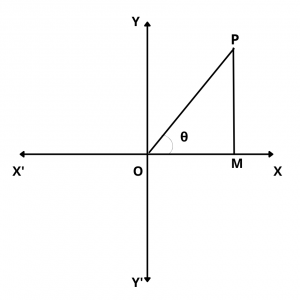# What is the General Solution of $$Sin \theta$$ = 0 ?

## Solution :

The general solution of $$sin \theta$$ = 0 is given by $$\theta$$ = $$n\pi$$, n $$\in$$ Z.

Proof :

We have,$$sin \theta$$ = $$PM\over OP$$

$$\therefore$$   $$sin \theta$$ = 0

$$\implies$$  $$PM\over OP$$ = 0

$$\implies$$ PM = 0

$$\implies$$  OP coincides with OX or OX’

$$\implies$$  $$\theta$$ = 0, $$\pi$$, $$2\pi$$, ….., $$-\pi$$, $$-2\pi$$, $$-3\pi$$, ….

$$\implies$$ $$\theta$$ = $$n\pi$$,  n $$\in$$ Z

Hence, $$\theta$$ = $$n\pi$$,  n $$\in$$ Z is the general solution of $$sin \theta$$ = 0.